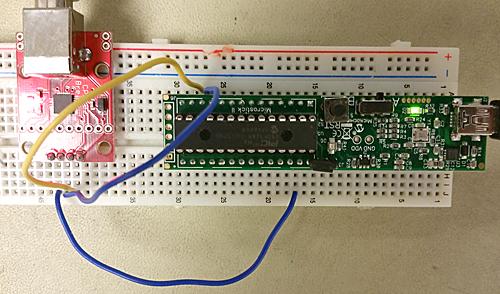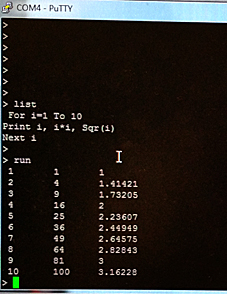Cornell University ECE4760
Interpreters

PIC32MX250F128B

Introduction

Sometimes it is useful to program directly on the PIC32. This can take the form of an interpreter.

1. MicroMite MkII on PIC32 by Geoff Graham at geoff@mmbasic.com
The 4.5 version at the bottom of this page can be downloaded directly to the PIC32MX250 and runs a version of Basic called MMBasic.
Just flash the hex file and hook a serial adaptor to pins 11 and 12, as described in the manual.
Images show the board and a 3-line program edited and running on the PIC32.2. IRIXBASIC on PIC32
http://www.andrewhazelden.com/blog/2012/07/now-presenting-irixbasic/
3. FORTH
4. MicroPython
https://github.com/micropython/micropython/wiki
5. PyMite and Python-on-a-chip
6. eLua
http://www.eluaproject.net/
7. PicoC and ReMix

Adam Dunkels' uBasic ported to PIC32.

It is useful to be able to write small programs in a scripting language. This example is an interpreter based on a C program written by Adam Dunkels, originally to implement, in his words: A really tiny and stupid BASIC interpreter, written in a few hours for the fun of it. (see http://www.sics.se/~adam/ubasic/).

I hacked it to be more of a tiny and stupid, block-oriented, bracket-delimited, language and ported it to the PIC32 in MPLABX/GCC. Although the port runs in the Protothreads header, I cannot currently get the interpreter to yield in any useful fashion because it uses reentrant code which breaks the threader. To make this language actually useful, you would need to modify it to have better variable names, arrays, and a scheme for handling PIC32 I/O in a better fashion than just pushing bytes into memory. I made a very simple interactive download utility so that source code (see example below) can be written on a PC editor, then sent to the microcontroller by pasting into the terminal window, and terminated with the `@` sign. The following two examples demonstrate that the recursive nesting of statements works correctly, that you can input/output variables values to/from C, and that it is easy to add PIC32 i/o controls like outputA below, which outputs bits to port A.

```print 'uBasic demo';print 'cmd line input=', a ;for i= 0 to 5 {    print 'i=', i ;    k = 0 ;   if i=2 {print 'if i=2'; } ;   while k<3 {for j=1 to 2 {print 'i*j+k=', i*j+k ;}; k=k+1; delay 10;};}; print 'done' ; end;@

# Enter 1=on 0=off -1=toggle on b cmd ;print 'cmd line input=', a ;i = 0;while i<50 {   outputA 1, a ;   delay 100 ;   i = i+1 ;};end; @```

The C program
The interpreter is a modified version of the programs on Adam Dunkel's site, and are properly acknowledged in the code. The demo C code puts the interpreter into a thread and controls execution by a command line serial interface. The command line interface has three commands: l lists the current uBasic program, b runs it, d puts the interface into a wait-state until you cut/paste the new program into the command window.
(Code, pt_cornell_1_3_1.h, ZIP of project, uBasic_examples)

uBasic Program structure

The following table shows the syntax of the existing statements. You can easily add your own statements.

• The term expr in the following is any arithmetic expression which may include the usual operators (```+, -, *, /, %, &, |```), variables and parentheses. The mod operator is `%`.
• The term relation is `expr1>expr2` or `expr1<expr2` or `expr1=expr2`.
• The term var is a variable name, which must currently be a single letter, `a-z`.
All values are 32-bit integer only.
• The term statement is any complete statement.

The more formal BNF notation for the language is:

• `<variable> ::= <letter>`
• `<number>`` ::= 0 to 32768`
• `<variable_value> ::= contents of <variable>`
• `<relation> ::= <expression> ("<" | ">" | "=") <expression>`
• ```<expression> ::= <term> {( "+" | "-" | "&" | "|" ) <term>}```
• `<term> ::= <factor>` ```{( "*" | "/" | "%" ) <factor>}```
• `<factor> ::= <number> | <variable_value> | "(" <expression>` `")"`
• `<statement_sequence> ::= <statement> { <statement> } `
• ```<statement> ::= <assignment_statement> | <if_statement> | <while_statement> | <print_statement> | <for_statement> | <peek_statement> | <poke_statement> | <delay_statement> | <comment statement>```
• `<comment statement>` ```::= "#" [<any characters except ";">] ";"```
• ```<assignment_statement> ::= <variable> "=" <expression> ";"```
• `<peek_statement> ````::= peek <expression> "," <variable>``` ` ";"`
• `<poke_statement> ````::= poke <expression> "," <expression>``` ` ";"`
• `<delay_statement>` `::= delay <expression>` ` ";"`
• <outputA_statment> ::= outputA ```<expression> "," <expression>``` ` ";"`
• ```<if_statement> ::= if <relation> "{" <statement_sequence> "}" [ else "{" <statement_sequence> "}" ] ";"```
• ```<while_statement> ::= while <relation> ````"{" <statement_sequence> "}"` ";"
• `<for_statement>` ```::= for <variable> "=" <expression> to <expression> ````"{" <statement_sequence> "}"` ";"

All statements are terminated with a semicolon. Spaces, tabs, newlines are all ignored. All program constructs must case-match the templates below.

`statement` `example`
`for var = expr to expr { statement; statment; ... } ;` `for i=1 to 10 { j=j+1; print j; } ;`
`while relation { statement; statment; ... } ;` `while i<10 { i=i+1; print 'i=',i; } ;`

```if relation { statement; statment; ... } else ````{ statement; statment; ... } `;

```if c<255 {print c; delay(500);} else {print 'no button';}; ```
`print expr, 'string', ... ;` `print 'hi there', i+1;`
`peek address, var ;` `peek 3+32, x; # peek address, variable;`
`poke`` address, expr ;` `poke 40, i+2; # poke address, value`
outputA bit_num, operation ; Sets, clears or toggles portA bits.
bit_num parameter is the sum of the values of the bits to toggle. example: bit_num=5 means that bits 0 and 2 are changed. operation takes values 0=clear, 1=set, -1=toggle
`delay expr ;` `delay 500; # milliseconds ;`
`# this is a comment statement ;` `# comment ; Upper case in the comment breaks the parser. dont know why yet.`
`var = expr ;` `a = (i+1)/j; # assignment ;`

To implement a new statement (in this example outputA above):

• In pt_cornell_1_3_1.h add the statement token name to
static const struct keyword_token keywords[]
as TOKENIZER_OUTPUTA
• Write the logic to parse and execute the statment. In the case of outputA above
static void outputA_statement(void){
int bits ;
int val ;
accept(TOKENIZER_OUTPUTA);
bits = expr() ;
mPORTASetPinsDigitalOut(bits); //Set port as output
accept(TOKENIZER_COMMA);
val = expr() ;
//printf("%d %d\n\r", bits, val);
if (val==0)
mPORTAClearBits(bits);
if (val==1)
mPORTASetBits(bits);
if (val==-1)
mPORTAToggleBits(bits);
accept(TOKENIZER_CR);
}

Where you:
• check for, then discard the statement token
• use the expr() function to get an expression, then designate the output bits
• check for, then discard the comma token
• get another expression, and set the output bits to one of three states
• check for, then discard the end-of-line token, which I set to be semicolon.
• Add the function name to the statement dispatch function void statement(void)
case TOKENIZER_OUTPUTA:
outputA_statement();
break;

Copyright Cornell University August 15, 2018